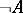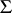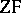# Mathematical logic

symbolic logic

The branch of mathematics concerned with the study of mathematical proofs and questions in the foundation of mathematics.

## Historical sketch.

The idea of constructing a universal language for the whole of mathematics, and of the formalization of proofs on the basis of such a language, was suggested in the 17th century by G. Leibniz. But not until the middle of the 19th century did there appear the first scientific work on the algebraization of Aristotelean logic (G. Boole, 1847, A. de Morgan 1858). After G. Frege (1879) and C. Peirce (1885) put the logic of predicates, variables and quantifiers into the language of algebra, it became possible to apply this language to questions in the foundations of mathematics.

On the other hand, the creation of non-Euclidean geometry in the 19th century strongly shook the confidence of mathematicians in the absolute reliability of the geometrical intuition on which Euclidean geometry was founded. Doubts about the reliability of geometrical intuition were also promoted as a result of the fact that in the development of infinitesimal calculus, mathematicians came across unexpected examples of everywhere-continuous functions without derivatives. The need appeared to separate the notion of a real number from the vague notion of a "variable" , which was based on geometric intuition. This problem was solved in various ways by K. Weierstrass, R. Dedekind and G. Cantor. They showed the possibility of "arithmetizing" analysis and function theory, as a result of which the arithmetic of integers came to be considered as the foundation of the whole of classical mathematics. Subsequently, the axiomatization of arithmetic was undertaken (Dedekind, 1888, and G. Peano, 1891). In this connection, Peano created a more suitable symbolic representation for the language of logic. Afterwards, this language was perfected in the joint work of B. Russell and A. Whitehead, Principia Mathematica (1910), in which they attempted to reduce the whole of mathematics to logic. But this attempt was not crowned with success, since it turned out to be impossible to deduce the existence of infinite sets from purely logical axioms. Although the logistic program of Frege–Russell on the foundations of mathematics never achieved its major aim, the reduction of mathematics to logic, in their papers they created a rich logical apparatus without which the appearance of mathematical logic as a valuable mathematical discipline would have been impossible.

At the turn of the 19th century into the 20th century, antinomies (cf. Antinomy) related to the fundamental ideas of set theory were found. The strongest impression at that time was made by the Russell paradox. Letbe the set containing exactly those sets which are not an element of itself. It is easy to convince oneself thatis an element of itself if and only ifis not an element of itself. Certainly one may circumvent this contradiction by stating that such a setcannot occur. However, if a set consisting precisely of all the elements satisfying some clearly defined condition, such as that given above in the definition of, need not exist, then where is the guarantee that in everyday work one will not meet with sets which also need not exist? And, in general, what conditions must the definition of a set satisfy so that the set does exist? One thing was clear: Cantor's theory of sets must somehow be restricted.

L.E.J. Brouwer (1908) opposed the application of rules of classical logic to infinite sets. In his intuitionistic program it was suggested that the abstraction of actual infinity be removed from the discussion, that is, remove infinite sets as complete collections. While admitting the existence of arbitrarily large natural numbers, the intuitionist comes out against the consideration of the natural numbers as a complete set. They believe that in mathematics every proof concerning the existence of an object must be constructive, that is, must be accompanied by a construction of that object. If the premise that the object sought for does not exist results in a contradiction, then this, in the opinion of intuitionists, cannot be considered as a proof of its existence. Particular criticism on the part of intuitionists is aimed at the law of the excluded middle. Since the law was initially considered in association with finite sets, and taking into account the fact that many properties of finite sets are not satisfied by infinite sets (for example, that each proper part is less than the whole), intuitionists regard the application of this law to infinite sets as inadmissible. For example, in order to assert that Fermat's problem has a positive or a negative solution, the intuitionist must give the corresponding solution. So long as Fermat's problem is unsolved, this disjunction is regarded as illegitimate. The same requirement is imposed on the meaning of each disjunction. This requirement of intuitionists may create difficulties even in the consideration of problems connected with finite sets. Imagine that, with eyes closed, a ball is taken from an urn in which there are three black and three white balls, and that the ball is then put back. If no one sees the ball, then one cannot possibly know what colour it was. However, it is doubtful whether one can seriously dispute the certainty of the assertion that the ball was either black or white in colour.

Intuitionists have constructed their own mathematics, with interesting distinctive peculiarities, but it has turned out to be more complicated and cumbersome than classical mathematics. The positive contribution of intuitionists to the investigation of questions in the foundations of mathematics is that they have once more decisively stressed the distinction between the constructive and the non-constructive in mathematics; they have made a careful analysis of the many difficulties which have been encountered in the development of mathematics, and, by the same token, they have contributed to overcoming them.

D. Hilbert (see the Appendices VII–X in ) planned another way to overcome the difficulties arising in the foundations of mathematics at the turn of the 19th century into the 20th century. This route, based on the application of the axiomatic method in the discussion of formal models of interesting mathematics, and in the investigation of questions of consistency of such models by reliable finitary means, was given the name Hilbert's finitism in mathematics. Recognizing the unreliability of geometrical intuition, Hilbert first of all undertook a careful review of Euclidean geometry, liberating it from the appeal to intuition. As a result of this revision his Grundlagen der Geometrie (1910) appeared, .

Questions of consistency of various theories were essentially considered even before Hilbert. Thus the projective model of the non-Euclidean Lobachevskii geometry constructed by F. Klein (1871) reduced the question of consistency of Lobachevskii's geometry to the consistency of Euclidean geometry. The consistency of Euclidean geometry can similarly be reduced to the consistency of analysis, that is, the theory of real numbers. However, it was not clear how it would be possible to construct models of analysis and arithmetic for these consistency proofs. The merit of Hilbert is that he gave a direct way for the investigation of this question. Consistency of a given theory means that one cannot obtain a contradiction in it, that is, it is not possible to prove both an assertionand its negation. Hilbert suggested representing the theory under discussion as a formal axiomatic system, in which those and only those assertions are derivable which are theorems of that theory. Then for the proof of consistency it suffices to establish the non-derivability in the theory of certain assertions. Thus a mathematical theory whose consistency one wishes to prove becomes an object of study in a mathematical science which Hilbert called metamathematics, or proof theory.

Hilbert wrote that the paradoxes of set theory have arisen not from the law of the excluded middle but "rather that mathematicians have used inadmissible and meaningless formations of ideas which in my proof theory are excluded …. To remove from mathematicians the law of the excluded middle is the same as taking the telescope away from astronomers or forbidding a boxer to use his fists" (see ). Hilbert suggested distinguishing between "real" and "ideal" assumptions of classical mathematics. The first have a genuine meaning but the second need not. Assumptions corresponding to the use of the actual infinite are ideal. Ideal assumptions can be added to the real ones in order that the simple results of logic be applicable even to arguments about infinite sets. This essentially simplifies the structure of the whole theory in much the same way as the addition of the line at infinity intersecting any two parallel lines in the projective geometry of the plane.

The program suggested by Hilbert for the foundation of mathematics, and his enthusiasm for it, inspired his contemporaries into an intensive development of the axiomatic method. Thus the formation of mathematical logic as an independent mathematical discipline is linked with Hilbert's initiative, at the start of 20th century, and the subsequent development of proof theory based on the logical language developed by Frege, Peano and Russell.

## The objective and fundamental branches of mathematical logic; relation to other areas of mathematics.

The objective of modern mathematical logic is diverse. First of all one must mention the investigation of logical and logico-mathematical calculi founded on classical predicate calculus. In 1930 K. Gödel proved the completeness theorem for predicate calculus, according to which the set of all valid purely logical assertions of mathematics coincides with the set of all derivable formulas in predicate calculus (see Gödel completeness theorem). This theorem showed that predicate calculus is a logical system on the basis of which mathematics can be formulated. Based on predicate calculus various logico-mathematical theories have been constructed (see Logico-mathematical calculus), representing the formalization of interesting mathematical theories: arithmetic, analysis, set theory, group theory, etc. Side-by-side with elementary theories (cf. Elementary theory), higher-order theories were also considered. In these one also admits quantifiers over predicates, predicates over predicates, etc. The traditional questions studied in these formal logical systems were the investigation of the structure of the deductions in the system, derivability of various formulas, and questions of consistency and completeness.

The Gödel incompleteness theorem on arithmetic, proved in 1931, destroyed the optimistic hopes of Hilbert for a complete solution of questions in the foundations of mathematics by the means mentioned above. According to this theorem, if a formal system containing arithmetic is consistent, then the assertion of its consistency expressed in the system cannot be proved by formalization within it. This means that with questions on foundations of mathematics the matter is not as simple as first desired or believed by Hilbert. But Gödel had already noted that the consistency of arithmetic could be proved by using fairly reliable constructive means, although still far from the means that are formalized in arithmetic. Similar proofs of the consistency of arithmetic were obtained by G. Gentzen (1936) and P.S. Novikov (1943) (cf. also Gentzen formal system).

As a result of the analysis of Cantor's set theory and the related paradoxes, various systems of axiomatic set theory were constructed with various restrictions on the formation of sets, to exclude the known inconsistencies. Within these axiomatic systems suitably extensive parts of mathematics could be developed. The consistency question for fairly rich axiomatic systems of set theory remains open. Of the most important results obtained in axiomatic set theory one must note Gödel's result on the consistency of the continuum hypothesis and the axiom of choice in the Bernays–Gödel system(1939), and the results of P. Cohen (1963) on the independence of these axioms from the Zermelo–Fraenkel axioms. One should note that these two axiom systems,and, are equiconsistent. For the proof of his result Gödel introduced the important idea of a constructible set (see Gödel constructive set) and proved the existence of a model ofconsisting of those sets. Gödel's method was used by Novikov for the proof of the consistency of certain other results in descriptive set theory (1951). For the construction of models of the set theoryin which the negation of the continuum hypothesis or the axiom of choice holds, Cohen introduced the so-called forcing method, which subsequently became a fundamental method for constructing models of set theory with various properties (cf. also Model theory).

One of the most remarkable achievements of mathematical logic was the development of the notion of a general recursive function and the formulation of the Church thesis, asserting that the notion of a general recursive function makes precise the intuitive notion of an algorithm. Of the equivalent elaborations of the notion of an algorithm the most widely used are the idea of a Turing machine and a Markov normal algorithm. In essence all mathematics is connected with some algorithm or other. But the possibility of identifying undecidable algorithmic problems (cf. Algorithmic problem) in mathematics appeared only with the refinement of the notion of an algorithm. Undecidable algorithmic problems were discovered in many areas of mathematics (algebra, number theory, topology, probability theory, etc.). Moreover, it turned out that they could be connected with very widespread and fundamental ideas in mathematics. Research into algorithmic problems in various areas of mathematics, as a rule, is accompanied by the penetration of the ideas and methods of mathematical logic into the area, which then leads to the solution of other problems no longer of an algorithmic nature.

The development of a precise notion of an algorithm made it possible to refine the notion of effectiveness and to develop on that basis a refinement of the constructive directions in mathematics (see Constructive mathematics), which embodies certain features of intuitionism, but is essentially different from the latter. The foundations of constructive analysis, constructive topology, constructive probability theory, etc., were laid.

In the theory of algorithms itself it is possible to pick out research in the domain of recursive arithmetic, containing various classifications of recursive and recursively-enumerable sets, degrees of undecidability of recursively-enumerable sets, research into the complexity of description of algorithms and the complexity of algorithmic calculations (in time and extent, see Algorithm, computational complexity of an; Algorithm, complexity of description of an). An extensively developing area in the theory of algorithms is the theory of enumeration.

As noted above, the axiomatic method exerted a major influence on the development of many areas of mathematics. Of special significance was the penetration of this method into algebra. Thus at the junction of mathematical logic and algebra, the general theory of algebraic systems (cf. Algebraic system), or model theory, arose. The foundations of this theory were laid by A.I. Mal'tsev, A. Tarski and their followers. Here one should note research into the elementary theory of classes of models, in particular, decidability questions in these theories, axiomatizability of classes of models, isomorphism of models, and questions of categoricity and completeness of classes of models.

An important place in model theory is occupied by studies on non-standard models of arithmetic and analysis. Even at the dawn of differential calculus, in the work of Leibniz and I. Newton, infinitely-small and infinitely-large quantities were regarded as numbers. Later the notion of a variable quantity appeared, and mathematicians turned away from the use of infinitely-small numbers, the modulus of which was different from zero and less than any positive real number, since their use required the loss of the Archimedean axiom. Only after three centuries, as a result of the development of the methods of mathematical logic, was it possible to establish that (non-standard) analysis with infinitely-small and infinitely-large numbers is consistent relative to the usual (standard) analysis of real numbers (cf. Non-standard analysis).

One could not conclude without mentioning the influence of the axiomatic method on intuitionistic mathematics. Thus, as long ago as 1930, A. Heyting introduced formal systems of intuitionistic logic of propositions and predicates (constructive propositional and predicate calculi). Later, formal systems of intuitionistic analysis were introduced (see, for example, ). Much of the research in intuitionistic logic and mathematics is concerned with formal systems. Special study was made of so-called intermediate logics (cf. Intermediate logic; also called super-intuitionistic logics), that is, logics lying between classical and intuitionistic logics. The notion of Kleene realizability of formulas is an attempt to interpret the idea of intuitionistic truth from the point of view of classical mathematics. However, it turned out that not every realizable formula of propositional calculus was derivable in intuitionistic (constructive) propositional calculus.

Modal logic has also been formalized. However, in spite of the large number of papers on formal systems of modal logic and its semantics (Kripke models), this can still be said to be an accumulation of uncoordinated facts.

Mathematical logic has a more applied value too; with each year there is a deeper penetration of the ideas and methods of mathematical logic into cybernetics, computational mathematics and structural linguistics.

See also the references to the articles on the various branches of mathematical logic.